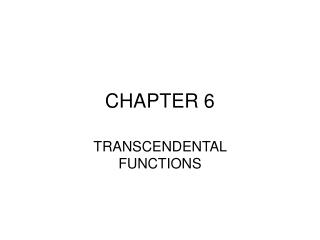DownloadDownload PresentationCHAPTER 6

# CHAPTER 6

Download Presentation## CHAPTER 6

- - - - - - - - - - - - - - - - - - - - - - - - - - - E N D - - - - - - - - - - - - - - - - - - - - - - - - - - -
##### Presentation Transcript

1. CHAPTER 6 TRANSCENDENTAL FUNCTIONS

2. 6.1 Natural Logarithm Function • The natural logarith function, denoted by ln, is defined by • The domaian of the natural logarithm function is the set of positive real numbers.

3. Geometric Meaning • Consider the graph of f(x) = 1/x. The ln(x) represents the area under f(x) between 1 and x.

4. Use First Fundamental Theorem to find the Derivative of the Natural Logarithm Function

5. Properties of natural logarithms • If a and b are positive numbers and r is any rational number, then • A) ln 1 = 0 • B) ln ab = ln a + ln b • C) ln(a/b) = ln a – ln b • D)

6. Logarithmic differentiation • Using properties of logarithms, a complicated expression can be rewritten as a sum or difference of less complicated expressions. Then, the function can be differentiated more easily. • Example next slide.

7. Find g’(x)

8. 6.2 Inverse Functions & Their Derivatives • If f is strictly monotonic on its domain, f has an inverse.

9. Inverse Function Theorem • Let f be differentiable and strictly monotonic on an interval I. If f’(x) does NOT euqal 0 at a certain x in I, then the inverse of f is differentiable at the corresponding point y = f(x) in the range of f and

10. Graphical interpretation • The slope of the tangent to a curve at point (x,y) is the reciprocal of the slope of the tangent to the curve of the inverse function at (y,x).

11. 6.3 The Natural Exponential Function • The inverse of ln is called the natural exponential function and is denoted by exp. Thus x = exp y, and y = ln x. • The letter “e” denotest he unique positive real number such than ln e = 1.

12. The natural exponential function and the natural logarithmic function are inverses of each other. • Properties that apply to inverse functions apply to these 2 functions.

13. The derivative of the natural exponential function is itself

14. Integrate the following:

15. 6.4 General Exponential & Logarithmic Functions

16. Logarithms could have a base other than e.

17. Example:

18. 6.5 Exponential Growth & Decay • Functions modeling exponential growth (or decay) are of this form:

19. Compound InterestA = amountr = interest raten = # times compoundedt=time in years

20. 6.6 1st-Order Linear Differential Equations • Sometimes it is not possible to separate an equation such that all expressions with x and dx are on one side and y and dy are on the other. • General form of a first-order linear differential equation:

21. 6.7 Approximations for Differential Equations Slope fields: Consider a first-order differential equation of the form y’ = f(x,y) At the point (x,y) the slope of a solution is given by f(x,y). Example:y’ = 3xy, at (2,4), y’=24, at (-2,1), y’=-6; at (0,5), y’ = 0; at (2,0), y’=0, etc. If all the slopes (y’) were graphed on a coordinate axes at those specific points, the resulting graph would be a “slope field”.

22. Approximating solutions of a differential equation • Euler’s Method: To approximate the folution of y’ = f(x,y) with initial condition y(x-not)=y-not, choose a step size ha nd repeat the following steps for n = 1,2,3,…

23. Applying Euler’s Method • Use your calculator and the table function to evaluate the function until the solution is found with the desired error.

24. 6.8 Inverse Trigonometric Functions & Their Derivatives • If the domain of the trigonometric functions is restricted, a portion of the curve is monotonic and has an inverse.

25. Using triangles, some useful identities are established.

26. Derivatives of 4 Inverse Trigonometric Functions

27. Example

28. 6.9 Hyperbolic Functions & Their Inverses

29. Derivatives of Hyperbolic Functions

30. Example